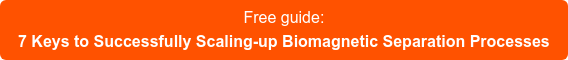Blog

Magnetic nanoparticles are susceptible to a magnetic field. If the magnetic field is constant then the particles will experience torque and will spin in place

Τ = m x BWhere τ is the torque, m is the magnetic moment of the particle, and B is the magnetic field.

If the magnetic field is not constant and changes over distance then it is said to have a gradient. If a magnetic particle is placed into a magnetic field gradient the particle will move in the direction of the gradient towards the place where the magnetic field is the strongest.Where FMis the force experienced by the particle with magnetic moment m, in magnetic field B.

The small spherical nanoparticles are suspended in fluid and thus experience a drag force as they move through the fluid. The drag force is dependent on particle radius r, fluid viscosity η, and fluid velocity ν.A research group in the chemical engineering department at Auburn University attempted to simulate the interactions of drag force and magnetic force on single magnetic particles. The goal was to develop a method to separate magnetic nanoparticles between 50 and 400 nm into groups of similar size with narrower distributions. They simulated the separation in Matlab. The simulation set-up consisted of individual particles injected into a capillary tube running parallel to a permanent magnet. Fluid flowed at a constant velocity through the tube and out a y-shaped junction. One fork of the y is closer to the magnet than the other. Predictive equations of force balance were used to determine the particle trajectories. The simulation predicted that the particles could be separated based on diameter. However, when the method was tested experimentally it failed to separate the particles by size. The separated solutions were found to have the same size distributions as the original sample.

The theory of the particle trajectories due to magnetic force and drag force was a good one, but it failed to account forparticle-particle interactions. Magnetic particles are capable of aggregating together. This interaction made it impossible to separate particles individually. It is important to keep this behavior in mind while using magnetic nanoparticles for separation protocols.

Exploiting Size-Dependent Drag and Magnetic Forces for Size-Specific Separation of Magnetic Nanoparticles. Hunter B. Rogers, TareqAnani, Young Suk Choi, Ronald J. Beyers, and Allan E. David. Int. J. Mol. Sci.2015, 16(8).

Related news: